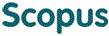### BROWSE

450 282

Cited 0 times in한국인에서 미맹출 견치와 소구치의 근원심 폭경 예측을 위한 최적의 치아조합

Other Titles
The best teeth combination to predict mesiodistal diameters ofthe unerupted canine and premolars of Koreans
Authors
김소화  ;  김성오  ;  이제호  ;  최병재  ;  최형준
Citation
Journal of the Korean Academy of Pediatric Dentistry (대한소아치과학회지), Vol.34(3) : 430-437, 2007
Journal Title
Journal of the Korean Academy of Pediatric Dentistry(대한소아치과학회지)
ISSN
1226-8496
Issue Date
2007
Abstract
The probability table of Moyers and prediction equation of Tanaka and Johnston that have been the most frequently used, cannot produce accurate prediction when used in Korean because they are based on the Caucasian popularity of the Northern European race. The method of Moyers or Tanaka and Johnston predicts sizes of the unerupted canine and premolars on the basis of the sizes of mandibular incisors. However, some of the recent papers raise a question as to whether the mandibular incisors are the best combination to predict the sizes of the unerupted canine and premolars. The purpose of this study is to determine which sum or combination of sums of permanent tooth widths present the best prediction for the unerupted canine and premolars in a Korean sample, to calculate a specific linear regression equation for this population, and to evaluate the clinical significance. A new linear regression equation was calculated based on the data of 178 Korean young adults(70 women, 108 men, mean age 21.63 years) with complete permanent dentitions. Fifty three more children(28 girls, 25 boys, mean age 14.22 years) were used as a validation sample for the application of the multiple linear regression equation. The conclusions were as follows: 1. The combination of the sums of permanent upper central incisors, lower lateral incisors and upper first molars was the best predictor for the unerupted canine and premolars in this sample($r=0.65{\sim}0.80$). 2. The multiple linear regression equation was calculated including sex and arch as additional predictor variables. male, upper: $Y\;=\;0.332{\times}X_0\;+\;6.195$ male, lower: $Y\;=\;0.332{\times}X_0\;+\;5.269$ female, upper: $Y\;=\;0.332{\times}X_0\;+\;5.929$ female, lower: $Y\;=\;0.332{\times}X_0\;+\;5.003$. The determination coefficient of the equation was 64% and a standard error of the estimate was 0.71mm. 3. In about 97% of the validation sample, the estimation of the tooth width sums of unerupted canine and premolars using the new multiple linear regression equation was smaller than 1mm compaired with the actual values.
Files in This Item:
Appears in Collections:
2. College of Dentistry (치과대학) > Dept. of Pediatric Dentistry (소아치과학교실) > 1. Journal Papers
Yonsei Authors
Kim, Seong Oh(김성오)https://orcid.org/0000-0002-8620-1377
Lee, Jae Ho(이제호)https://orcid.org/0000-0002-1556-3485
Choi, Byung Jai(최병재)
Choi, Hyung Jun(최형준)https://orcid.org/0000-0002-3315-6912
URI
https://ir.ymlib.yonsei.ac.kr/handle/22282913/97709
사서에게 알리기